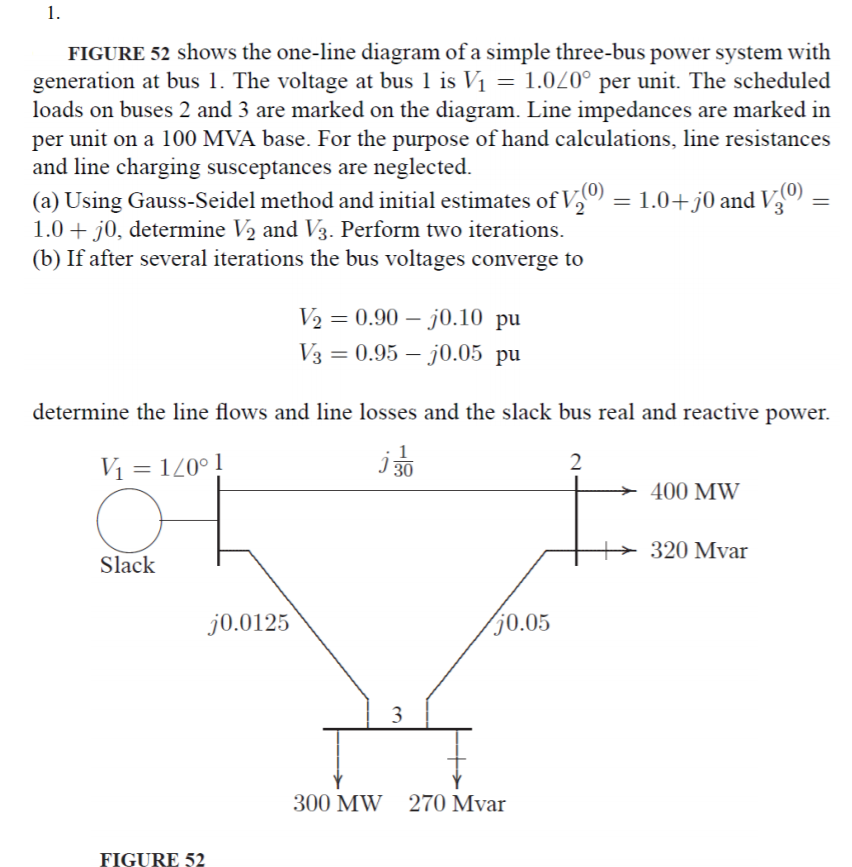# 1. FIGURE 52 shows the one-line diagram of a simple three-bus power system with generation at bus I. The voltage at bus l is V1 = 1.0L0° per unit. The scheduled loads on buses 2 and 3 are marked on the diagram. Line impedances are marked in per unit on a 100 MVA base. For the purpose of hand calculations, line resistances and line charging susceptances are neglected a) Using Gauss-Seidel method and initial estimates of Va 0)-1.0+)0 and V o)- ( 1.0 +j0, determine V2 and V3. Perform two iterations (b) If after several iterations the bus voltages converge to V20.90-j0.10 pu 0.95-70.05 pu determine the line flows and line losses and the slack bus real and reactive power. 2 400 MW 320 Mvar Slack 0.0125 0.05 300 MW 270 Mvar FIGURE 52

Questionhelp_outlineImage Transcriptionclose1. FIGURE 52 shows the one-line diagram of a simple three-bus power system with generation at bus I. The voltage at bus l is V1 = 1.0L0° per unit. The scheduled loads on buses 2 and 3 are marked on the diagram. Line impedances are marked in per unit on a 100 MVA base. For the purpose of hand calculations, line resistances and line charging susceptances are neglected a) Using Gauss-Seidel method and initial estimates of Va 0)-1.0+)0 and V o)- ( 1.0 +j0, determine V2 and V3. Perform two iterations (b) If after several iterations the bus voltages converge to V20.90-j0.10 pu 0.95-70.05 pu determine the line flows and line losses and the slack bus real and reactive power. 2 400 MW 320 Mvar Slack 0.0125 0.05 300 MW 270 Mvar FIGURE 52 fullscreen

### Want to see the step-by-step answer?